Research papers and code for "convolutional neural network":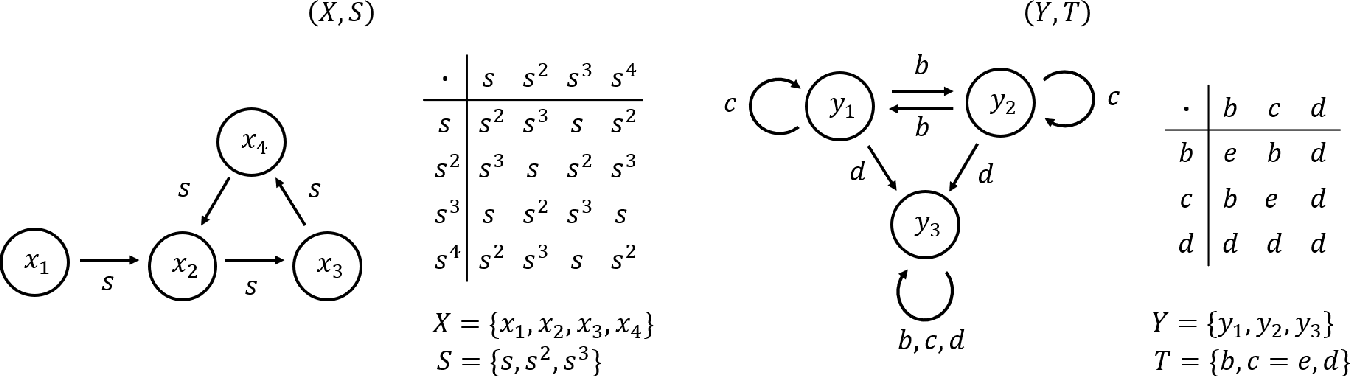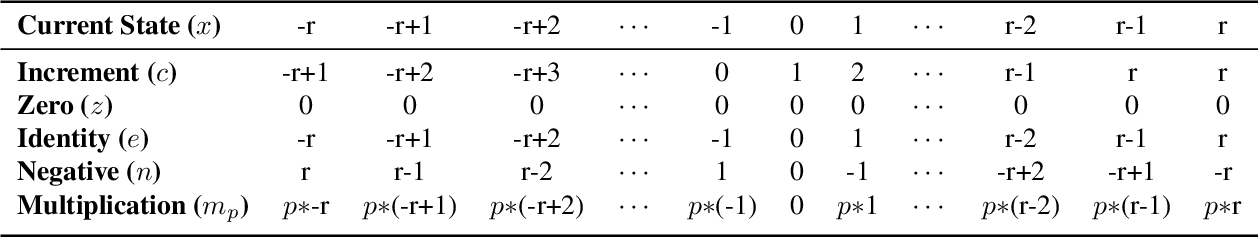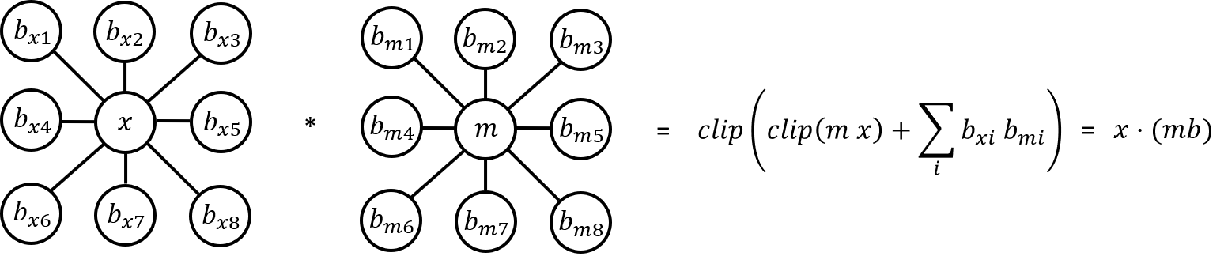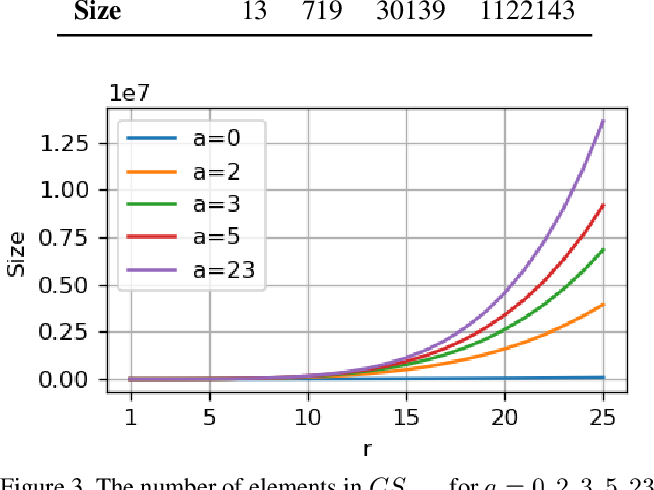Researchers are actively trying to gain better insights into the representational properties of convolutional neural networks for guiding better network designs and for interpreting a network's computational nature. Gaining such insights can be an arduous task due to the number of parameters in a network and the complexity of a network's architecture. Current approaches of neural network interpretation include Bayesian probabilistic interpretations and information theoretic interpretations. In this study, we take a different approach to studying convolutional neural networks by proposing an abstract algebraic interpretation using finite transformation semigroup theory. Specifically, convolutional layers are broken up and mapped to a finite space. The state space of the proposed finite transformation semigroup is then defined as a single element within the convolutional layer, with the acting elements defined by surrounding state elements combined with convolution kernel elements. Generators of the finite transformation semigroup are defined to complete the interpretation. We leverage this approach to analyze the basic properties of the resulting finite transformation semigroup to gain insights on the representational properties of convolutional neural networks, including insights into quantized network representation. Such a finite transformation semigroup interpretation can also enable better understanding outside of the confines of fixed lattice data structures, thus useful for handling data that lie on irregular lattices. Furthermore, the proposed abstract algebraic interpretation is shown to be viable for interpreting convolutional operations within a variety of convolutional neural network architectures.

* 9 pages
Click to Read Paper and Get Code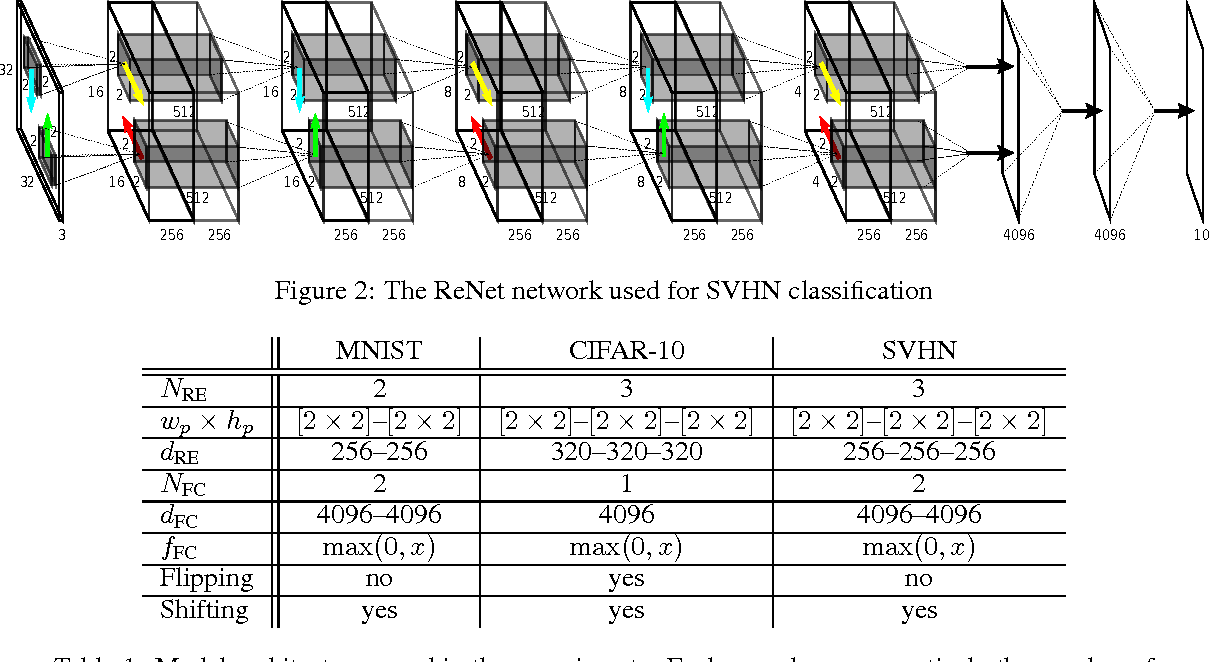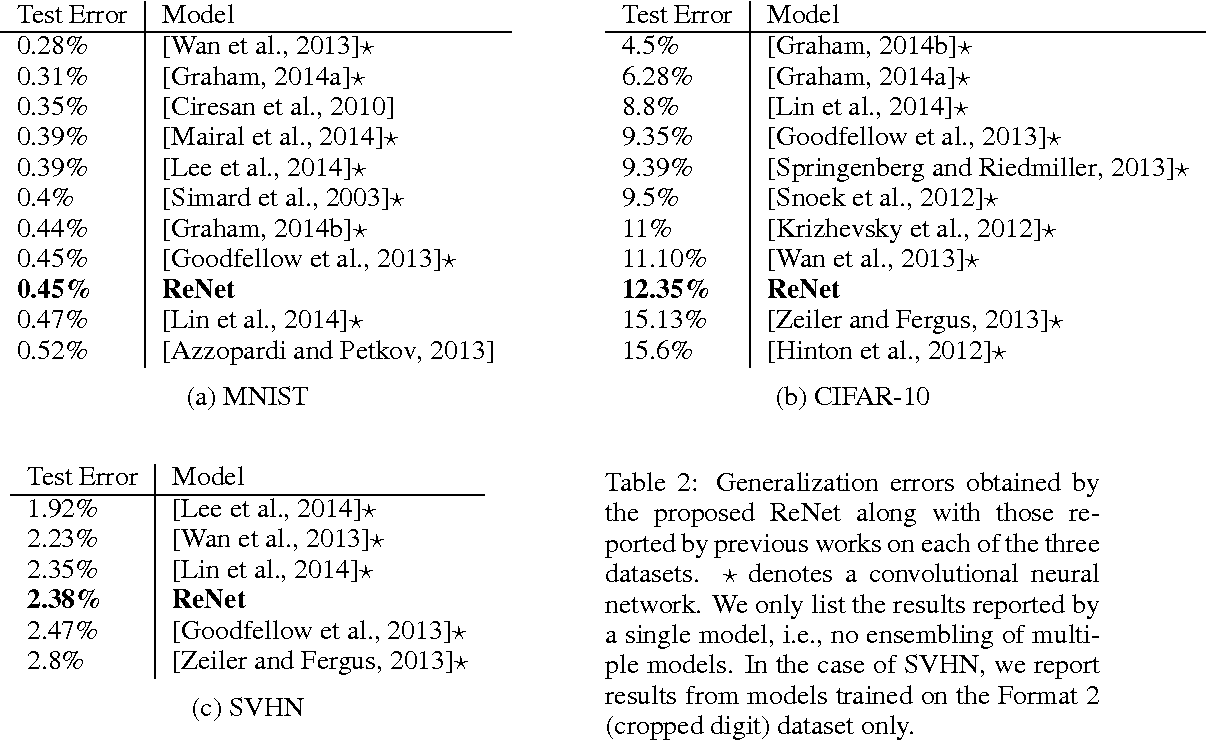In this paper, we propose a deep neural network architecture for object recognition based on recurrent neural networks. The proposed network, called ReNet, replaces the ubiquitous convolution+pooling layer of the deep convolutional neural network with four recurrent neural networks that sweep horizontally and vertically in both directions across the image. We evaluate the proposed ReNet on three widely-used benchmark datasets; MNIST, CIFAR-10 and SVHN. The result suggests that ReNet is a viable alternative to the deep convolutional neural network, and that further investigation is needed.

Click to Read Paper and Get Code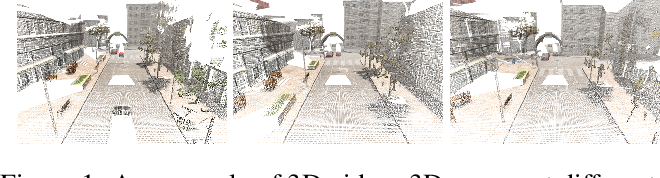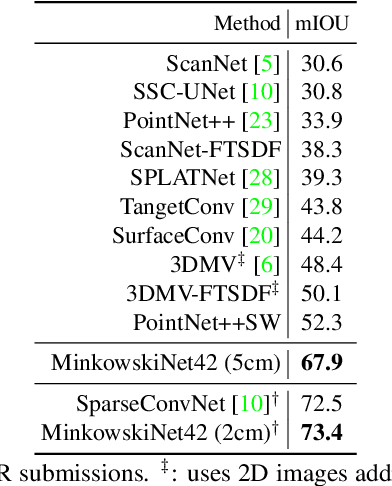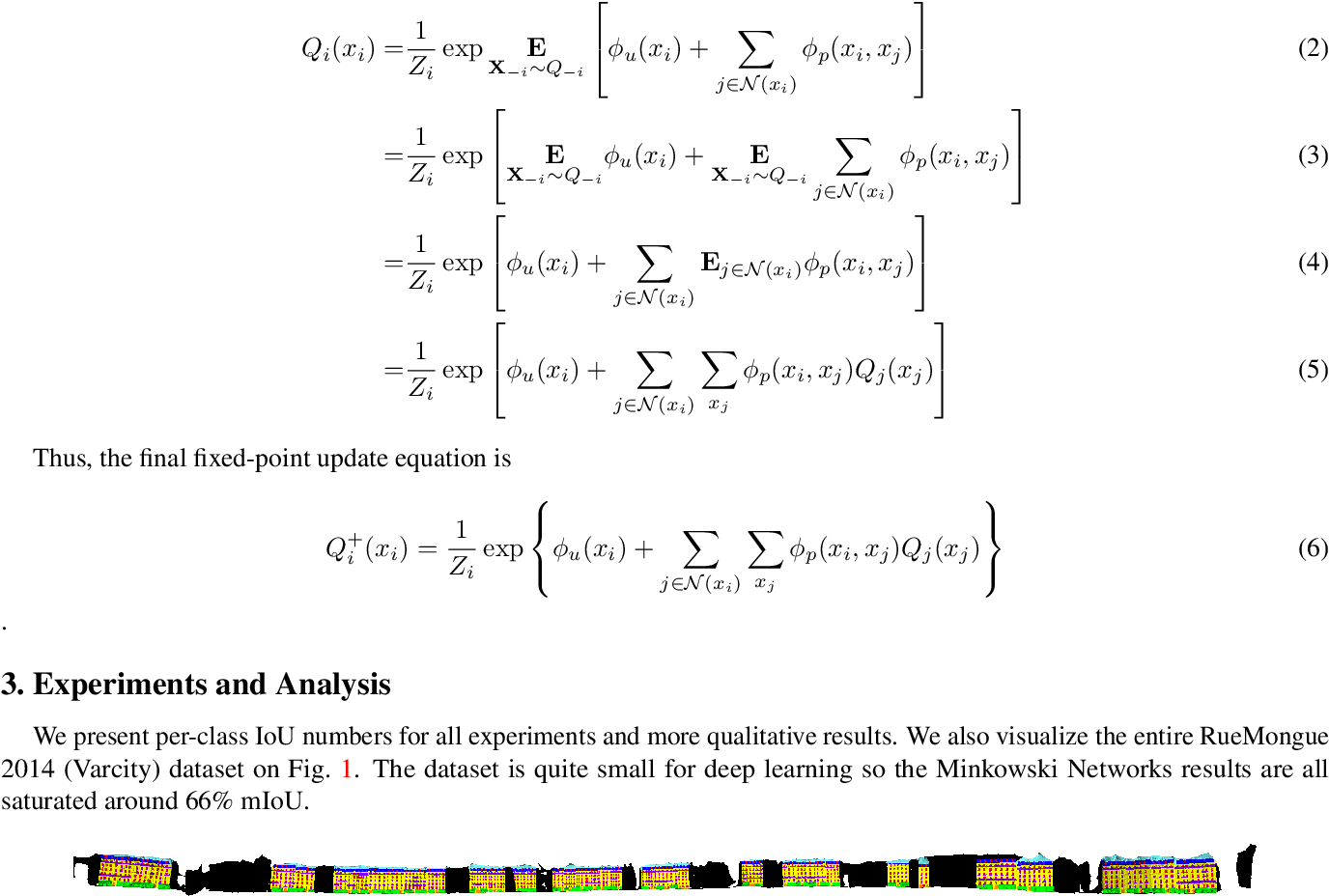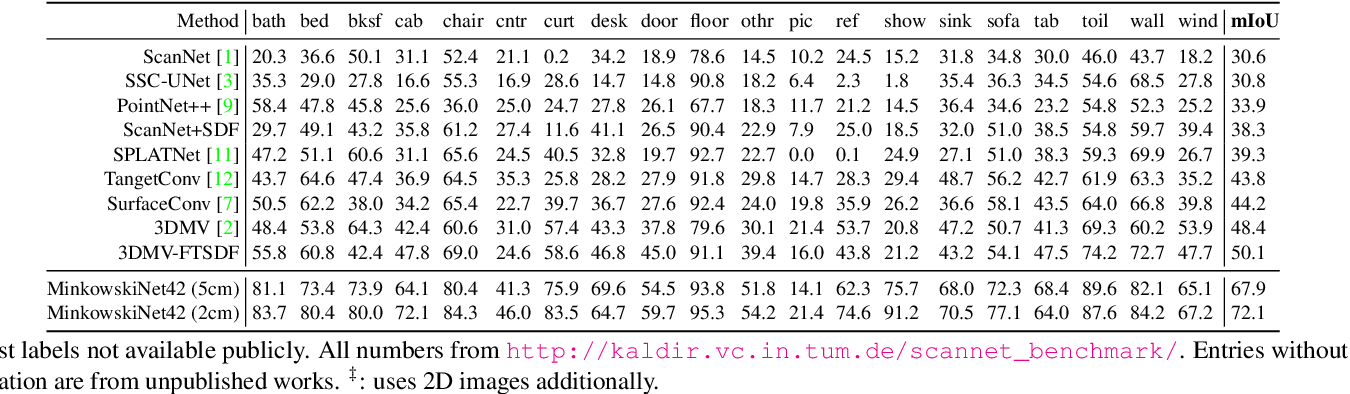In many robotics and VR/AR applications, 3D-videos are readily-available sources of input (a continuous sequence of depth images, or LIDAR scans). However, those 3D-videos are processed frame-by-frame either through 2D convnets or 3D perception algorithms. In this work, we propose 4-dimensional convolutional neural networks for spatio-temporal perception that can directly process such 3D-videos using high-dimensional convolutions. For this, we adopt sparse tensors and propose the generalized sparse convolution that encompasses all discrete convolutions. To implement the generalized sparse convolution, we create an open-source auto-differentiation library for sparse tensors that provides extensive functions for high-dimensional convolutional neural networks. We create 4D spatio-temporal convolutional neural networks using the library and validate them on various 3D semantic segmentation benchmarks and proposed 4D datasets for 3D-video perception. To overcome challenges in the 4D space, we propose the hybrid kernel, a special case of the generalized sparse convolution, and the trilateral-stationary conditional random field that enforces spatio-temporal consistency in the 7D space-time-chroma space. Experimentally, we show that convolutional neural networks with only generalized 3D sparse convolutions can outperform 2D or 2D-3D hybrid methods by a large margin. Also, we show that on 3D-videos, 4D spatio-temporal convolutional neural networks are robust to noise, outperform 3D convolutional neural networks and are faster than the 3D counterpart in some cases.

* CVPR'19
Click to Read Paper and Get Code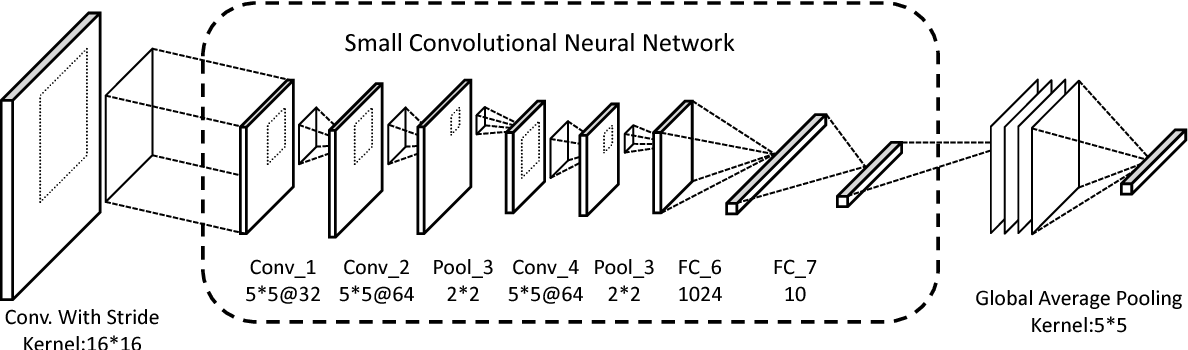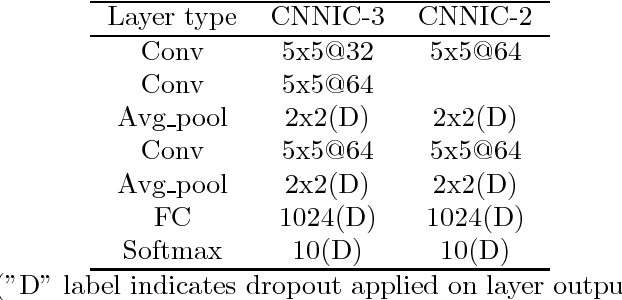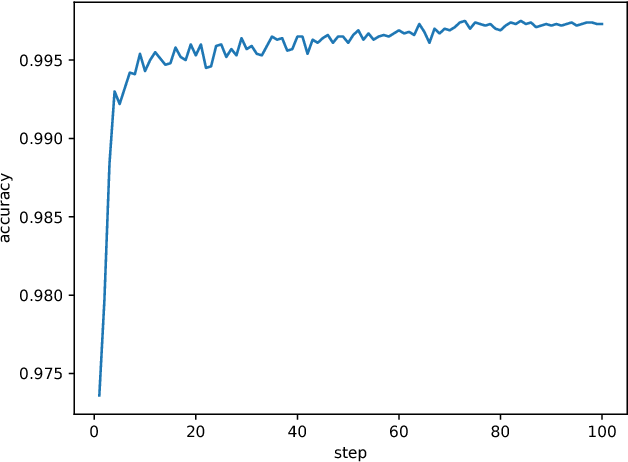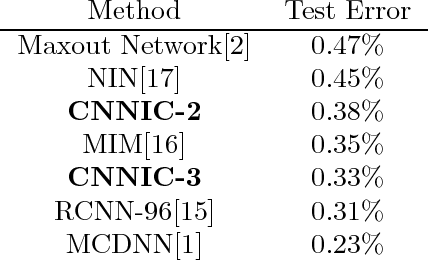Currently, increasingly deeper neural networks have been applied to improve their accuracy. In contrast, We propose a novel wider Convolutional Neural Networks (CNN) architecture, motivated by the Multi-column Deep Neural Networks and the Network In Network(NIN), aiming for higher accuracy without input data transmutation. In our architecture, namely "CNN In Convolution"(CNNIC), a small CNN, instead of the original generalized liner model(GLM) based filters, is convoluted as kernel on the original image, serving as feature extracting layer of this networks. And further classifications are then carried out by a global average pooling layer and a softmax layer. Dropout and orthonormal initialization are applied to overcome training difficulties including slow convergence and over-fitting. Persuasive classification performance is demonstrated on MNIST.

* 9 pages, 2 figures
Click to Read Paper and Get Code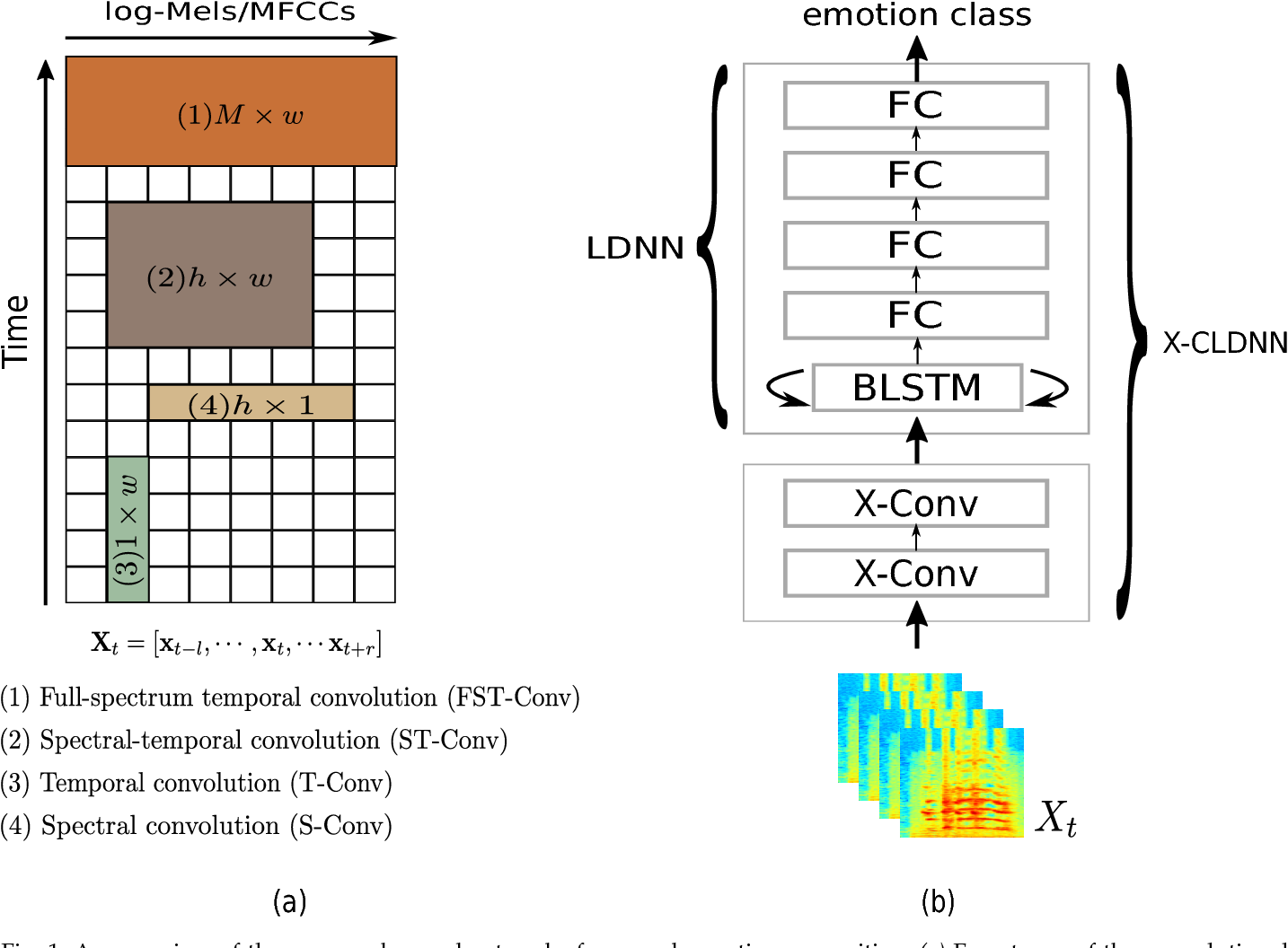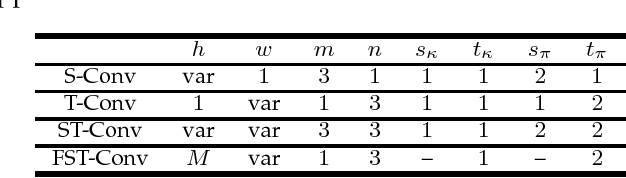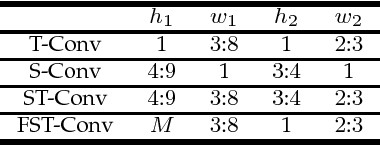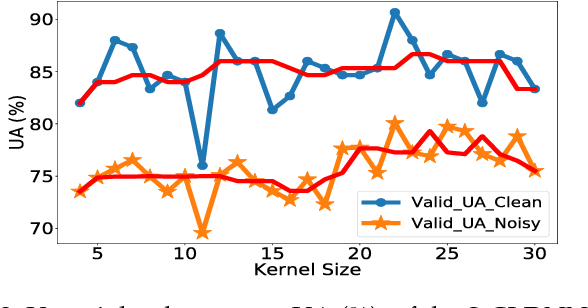Deep convolutional neural networks are being actively investigated in a wide range of speech and audio processing applications including speech recognition, audio event detection and computational paralinguistics, owing to their ability to reduce factors of variations, for learning from speech. However, studies have suggested to favor a certain type of convolutional operations when building a deep convolutional neural network for speech applications although there has been promising results using different types of convolutional operations. In this work, we study four types of convolutional operations on different input features for speech emotion recognition under noisy and clean conditions in order to derive a comprehensive understanding. Since affective behavioral information has been shown to reflect temporally varying of mental state and convolutional operation are applied locally in time, all deep neural networks share a deep recurrent sub-network architecture for further temporal modeling. We present detailed quantitative module-wise performance analysis to gain insights into information flows within the proposed architectures. In particular, we demonstrate the interplay of affective information and the other irrelevant information during the progression from one module to another. Finally we show that all of our deep neural networks provide state-of-the-art performance on the eNTERFACE'05 corpus.

* Revised Submission to IEEE Transactions
Click to Read Paper and Get Code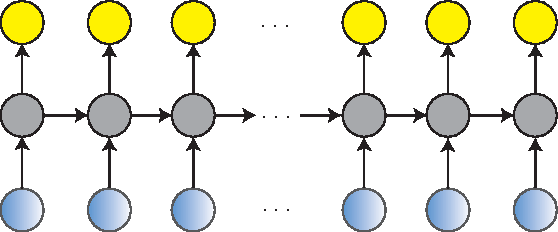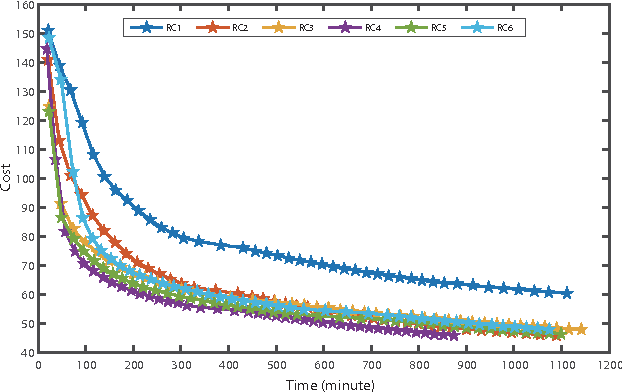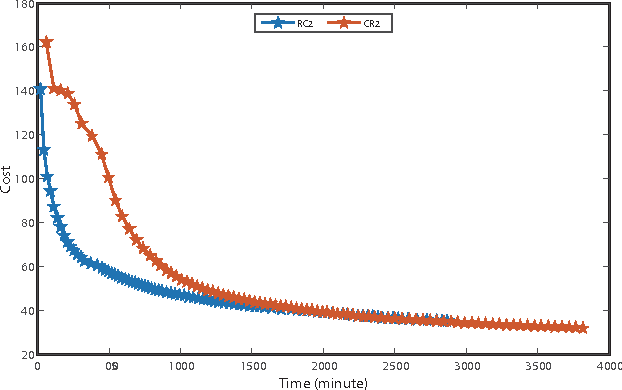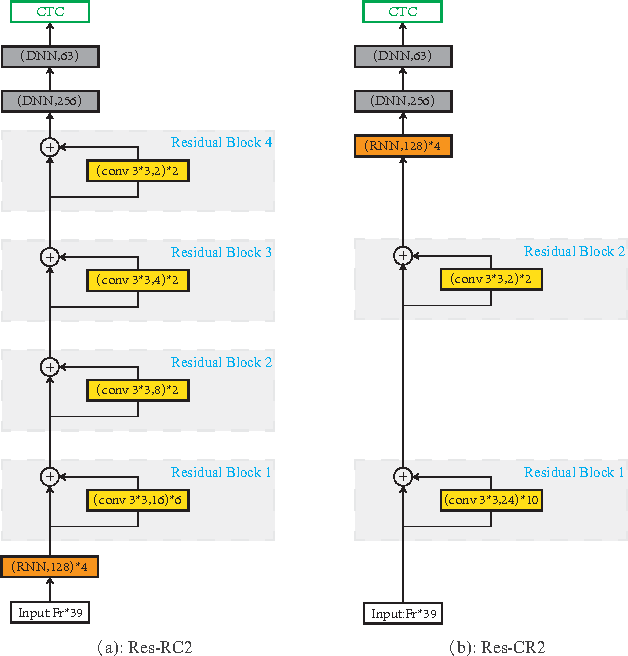A deep learning approach has been widely applied in sequence modeling problems. In terms of automatic speech recognition (ASR), its performance has significantly been improved by increasing large speech corpus and deeper neural network. Especially, recurrent neural network and deep convolutional neural network have been applied in ASR successfully. Given the arising problem of training speed, we build a novel deep recurrent convolutional network for acoustic modeling and then apply deep residual learning to it. Our experiments show that it has not only faster convergence speed but better recognition accuracy over traditional deep convolutional recurrent network. In the experiments, we compare the convergence speed of our novel deep recurrent convolutional networks and traditional deep convolutional recurrent networks. With faster convergence speed, our novel deep recurrent convolutional networks can reach the comparable performance. We further show that applying deep residual learning can boost the convergence speed of our novel deep recurret convolutional networks. Finally, we evaluate all our experimental networks by phoneme error rate (PER) with our proposed bidirectional statistical n-gram language model. Our evaluation results show that our newly proposed deep recurrent convolutional network applied with deep residual learning can reach the best PER of 17.33\% with the fastest convergence speed on TIMIT database. The outstanding performance of our novel deep recurrent convolutional neural network with deep residual learning indicates that it can be potentially adopted in other sequential problems.

* 11 pages, 13 figures
Click to Read Paper and Get Code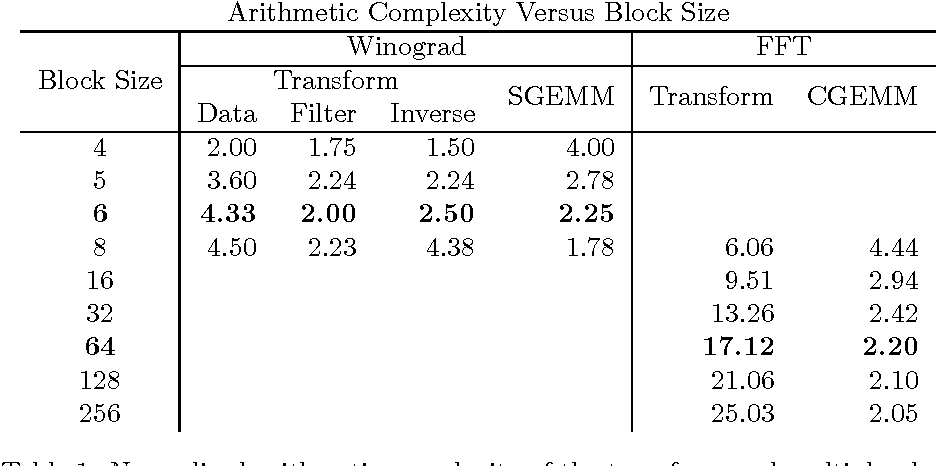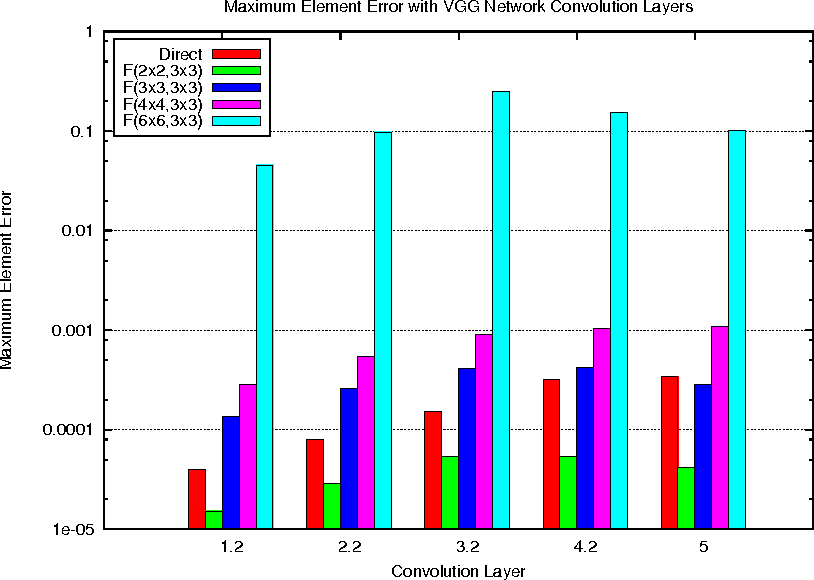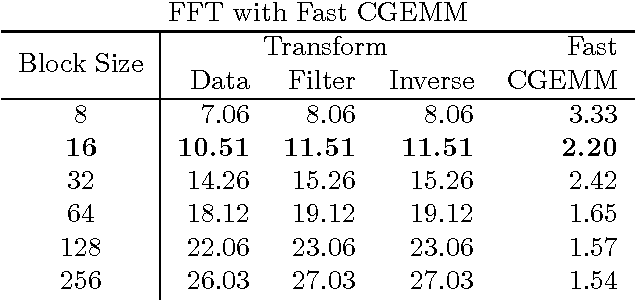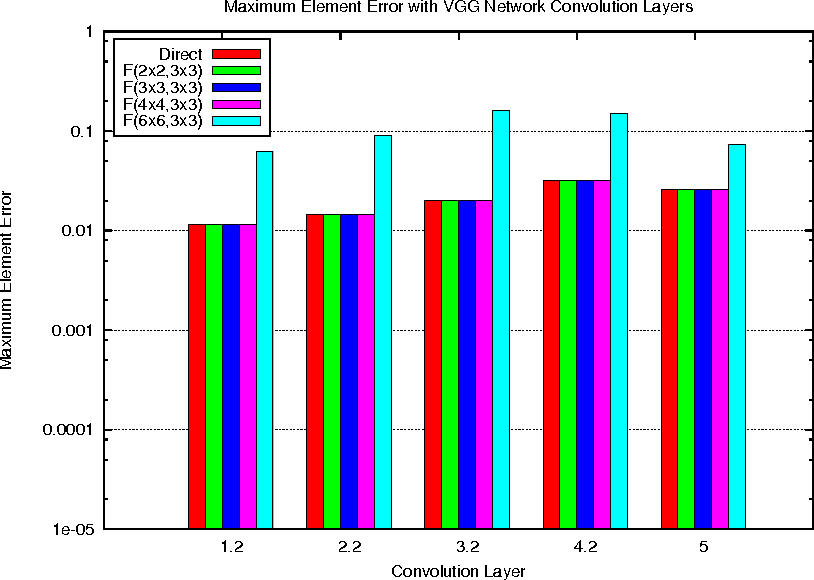Deep convolutional neural networks take GPU days of compute time to train on large data sets. Pedestrian detection for self driving cars requires very low latency. Image recognition for mobile phones is constrained by limited processing resources. The success of convolutional neural networks in these situations is limited by how fast we can compute them. Conventional FFT based convolution is fast for large filters, but state of the art convolutional neural networks use small, 3x3 filters. We introduce a new class of fast algorithms for convolutional neural networks using Winograd's minimal filtering algorithms. The algorithms compute minimal complexity convolution over small tiles, which makes them fast with small filters and small batch sizes. We benchmark a GPU implementation of our algorithm with the VGG network and show state of the art throughput at batch sizes from 1 to 64.

Click to Read Paper and Get Code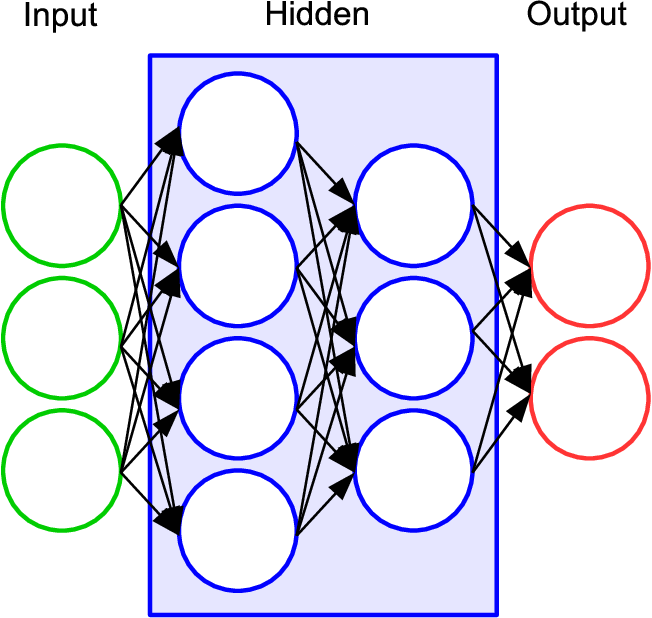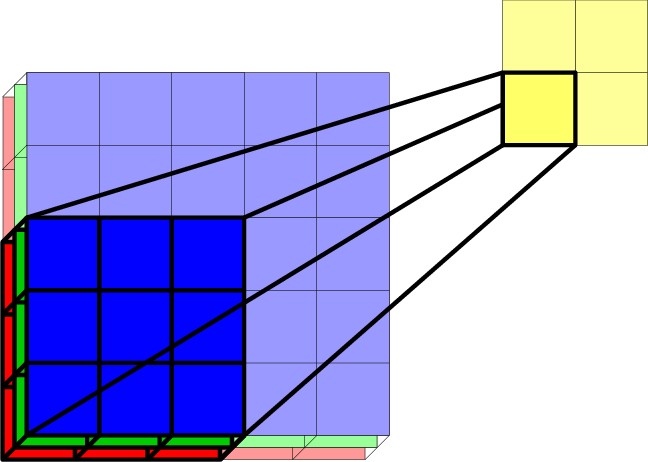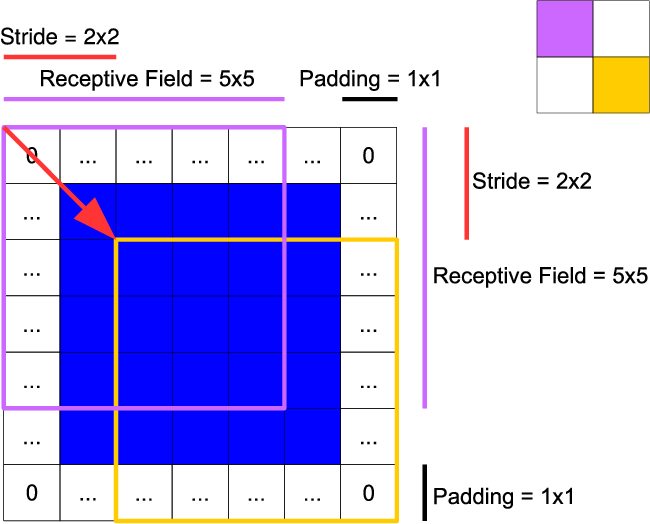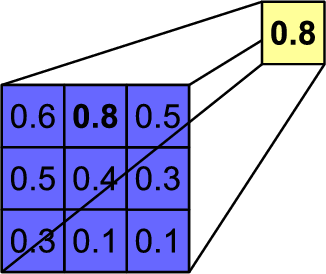Artificial neural networks have recently shown great results in many disciplines and a variety of applications, including natural language understanding, speech processing, games and image data generation. One particular application in which the strong performance of artificial neural networks was demonstrated is the recognition of objects in images, where deep convolutional neural networks are commonly applied. In this survey, we give a comprehensive introduction to this topic (object recognition with deep convolutional neural networks), with a strong focus on the evolution of network architectures. Therefore, we aim to compress the most important concepts in this field in a simple and non-technical manner to allow for future researchers to have a quick general understanding. This work is structured as follows: 1. We will explain the basic ideas of (convolutional) neural networks and deep learning and examine their usage for three object recognition tasks: image classification, object localization and object detection. 2. We give a review on the evolution of deep convolutional neural networks by providing an extensive overview of the most important network architectures presented in chronological order of their appearances.

* 17 pages (incl. references), 23 Postscript figures, uses IEEEtran
Click to Read Paper and Get Code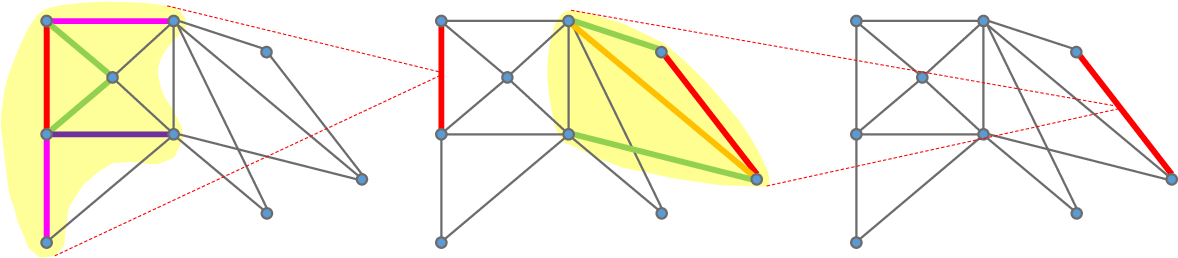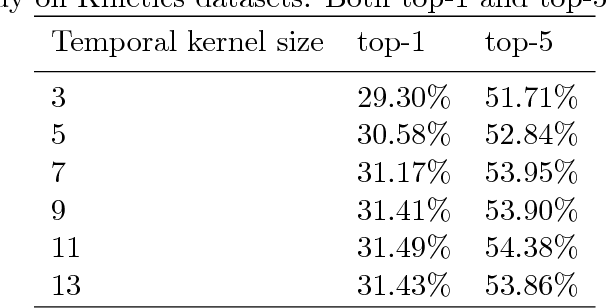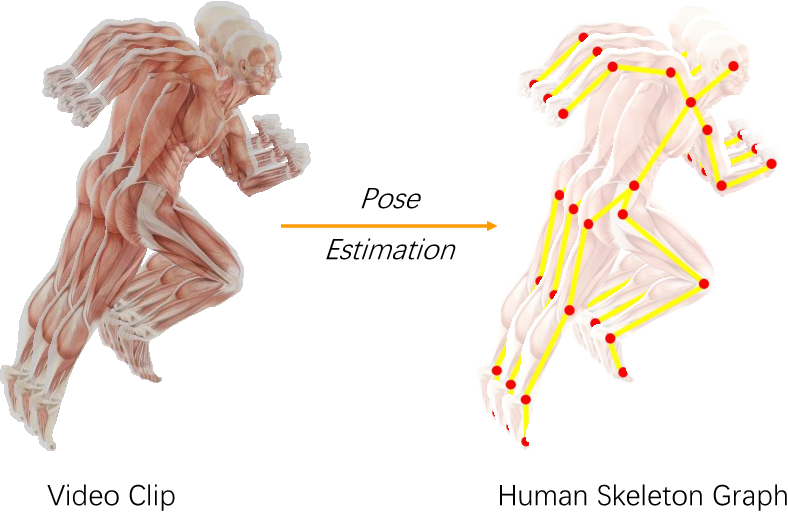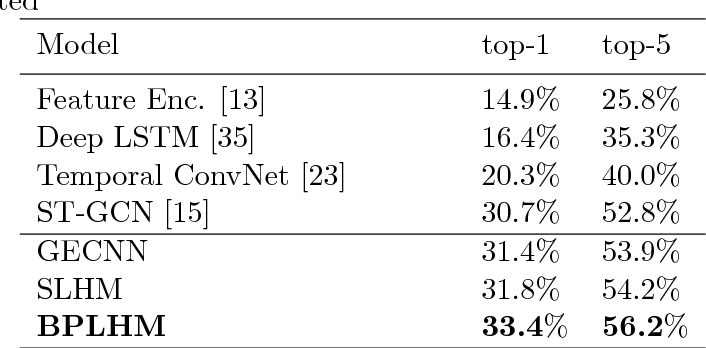This paper investigates body bones from skeleton data for skeleton based action recognition. Body joints, as the direct result of mature pose estimation technologies, are always the key concerns of traditional action recognition methods. However, instead of joints, we humans naturally identify how the human body moves according to shapes, lengths and places of bones, which are more obvious and stable for observation. Hence given graphs generated from skeleton data, we propose to develop convolutions over graph edges that correspond to bones in human skeleton. We describe an edge by integrating its spatial neighboring edges to explore the cooperation between different bones, as well as its temporal neighboring edges to address the consistency of movements in an action. A graph edge convolutional neural network is then designed for skeleton based action recognition. Considering the complementarity between graph node convolution and graph edge convolution, we additionally construct two hybrid neural networks to combine graph node convolutional neural network and graph edge convolutional neural network using shared intermediate layers. Experimental results on Kinetics and NTU-RGB+D datasets demonstrate that our graph edge convolution is effective to capture characteristic of actions and our graph edge convolutional neural network significantly outperforms existing state-of-art skeleton based action recognition methods. Additionally, more performance improvements can be achieved by the hybrid networks.

Click to Read Paper and Get Code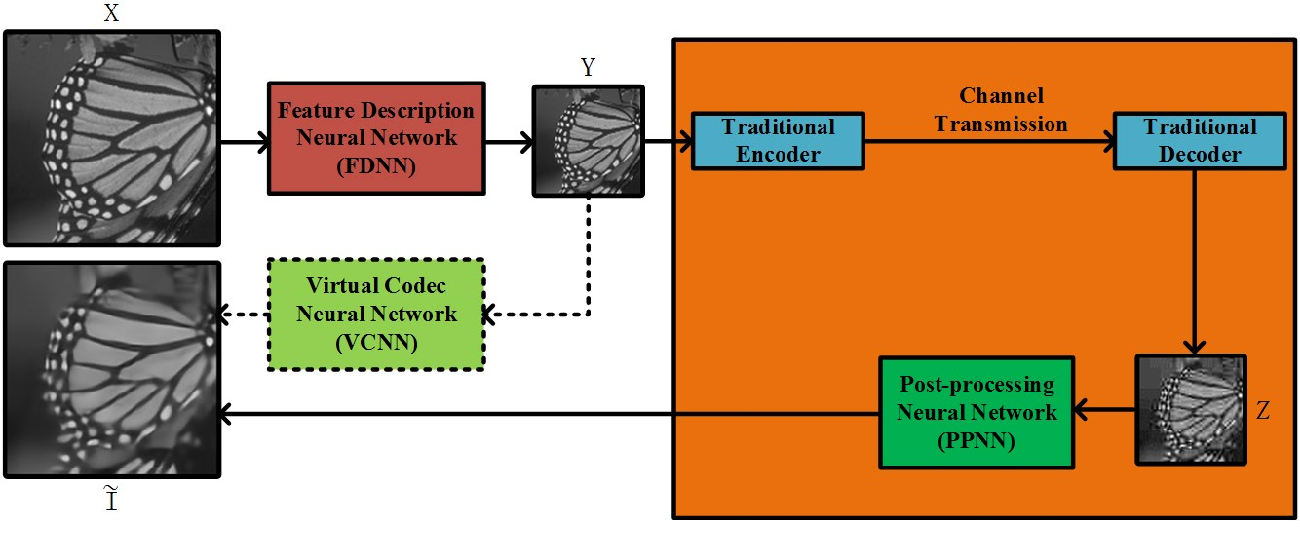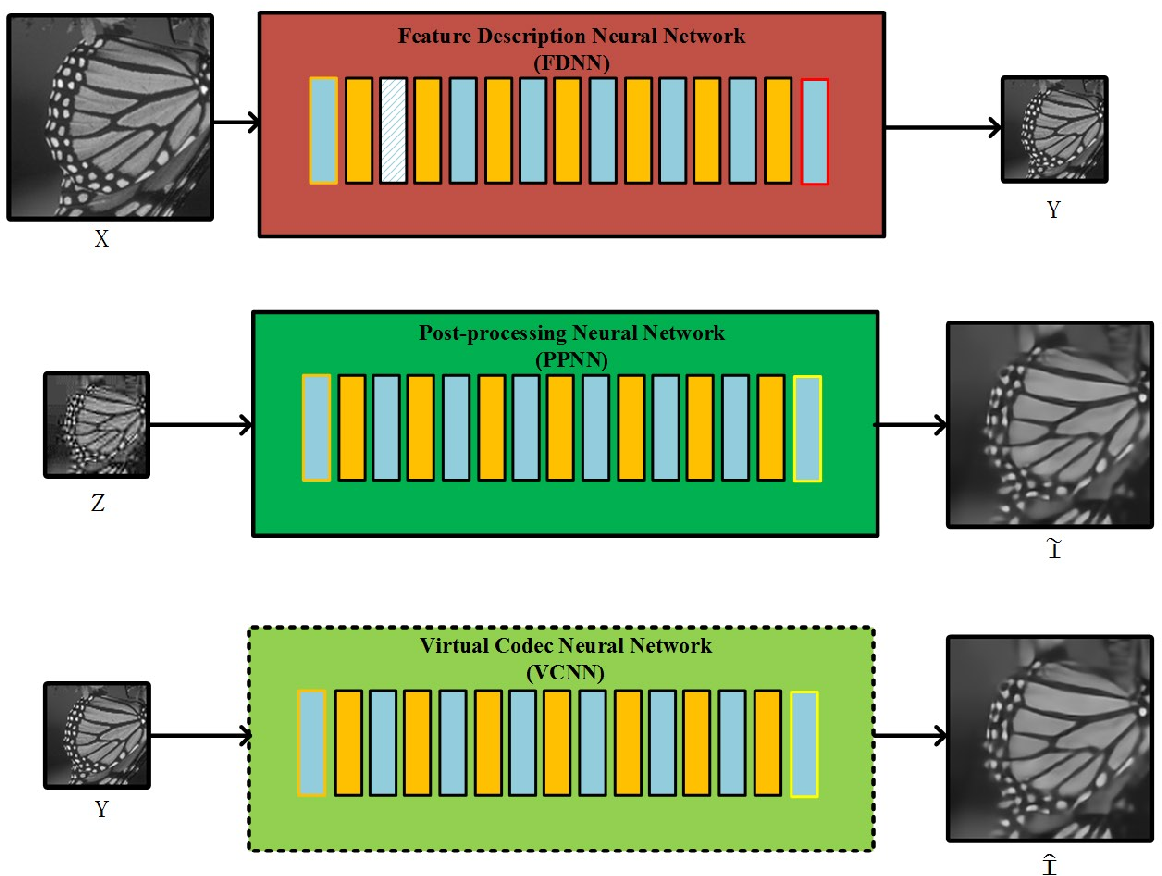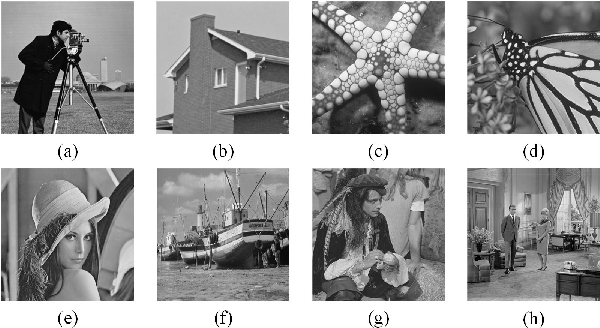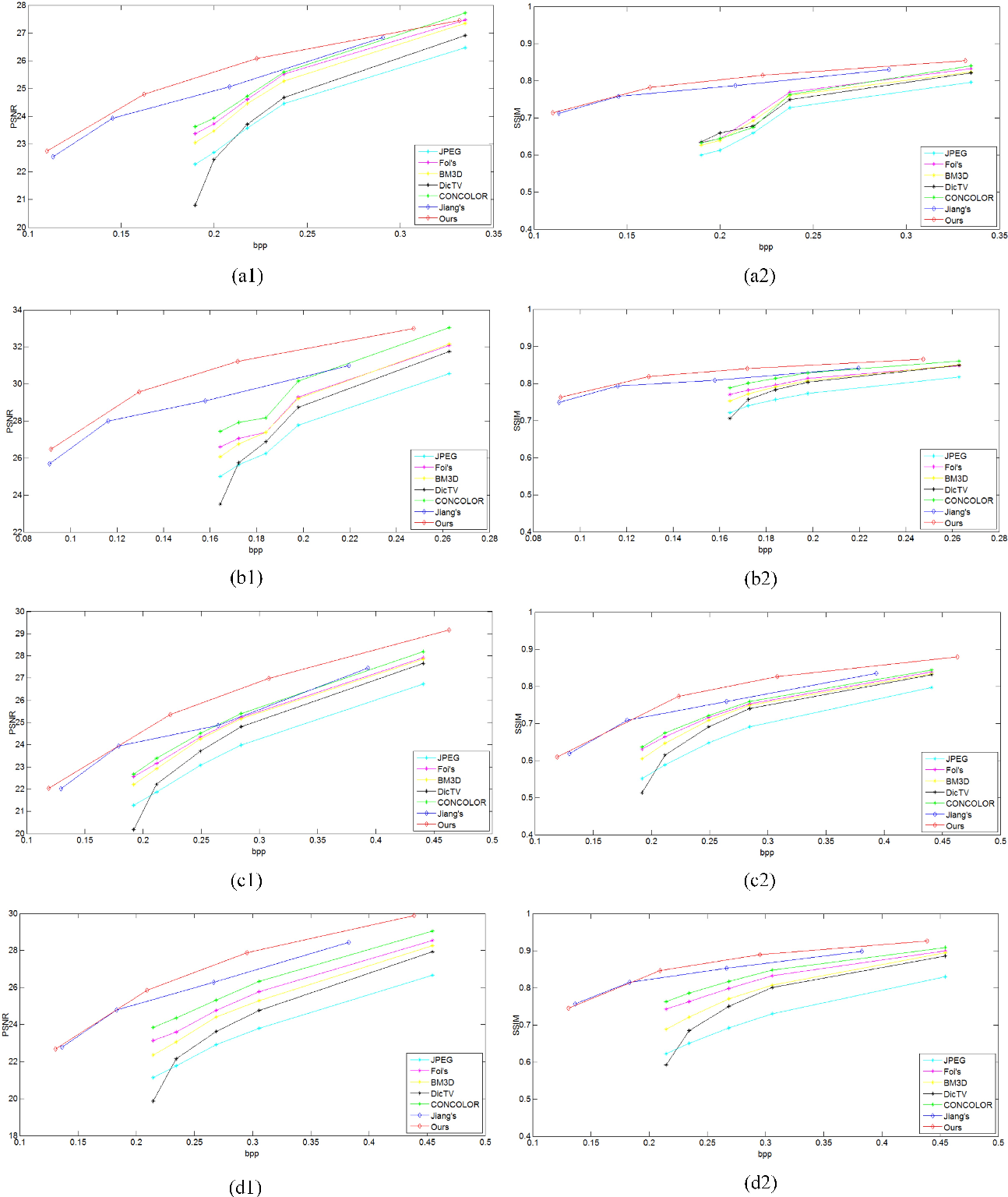Although deep convolutional neural network has been proved to efficiently eliminate coding artifacts caused by the coarse quantization of traditional codec, it's difficult to train any neural network in front of the encoder for gradient's back-propagation. In this paper, we propose an end-to-end image compression framework based on convolutional neural network to resolve the problem of non-differentiability of the quantization function in the standard codec. First, the feature description neural network is used to get a valid description in the low-dimension space with respect to the ground-truth image so that the amount of image data is greatly reduced for storage or transmission. After image's valid description, standard image codec such as JPEG is leveraged to further compress image, which leads to image's great distortion and compression artifacts, especially blocking artifacts, detail missing, blurring, and ringing artifacts. Then, we use a post-processing neural network to remove these artifacts. Due to the challenge of directly learning a non-linear function for a standard codec based on convolutional neural network, we propose to learn a virtual codec neural network to approximate the projection from the valid description image to the post-processed compressed image, so that the gradient could be efficiently back-propagated from the post-processing neural network to the feature description neural network during training. Meanwhile, an advanced learning algorithm is proposed to train our deep neural networks for compression. Obviously, the priority of the proposed method is compatible with standard existing codecs and our learning strategy can be easily extended into these codecs based on convolutional neural network. Experimental results have demonstrated the advances of the proposed method as compared to several state-of-the-art approaches, especially at very low bit-rate.

* 11 pages, 7 figures
Click to Read Paper and Get Code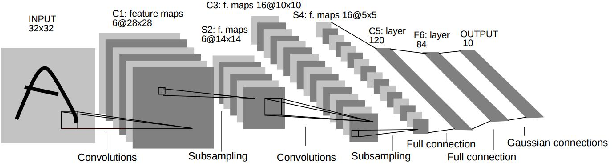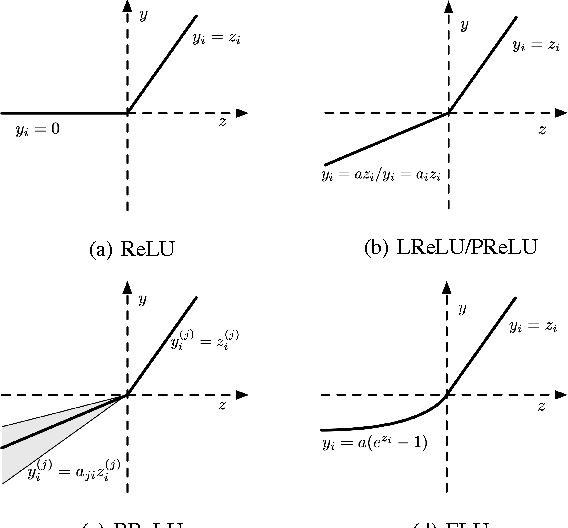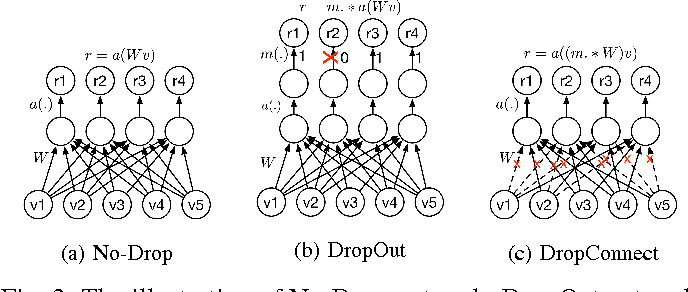In the last few years, deep learning has led to very good performance on a variety of problems, such as visual recognition, speech recognition and natural language processing. Among different types of deep neural networks, convolutional neural networks have been most extensively studied. Leveraging on the rapid growth in the amount of the annotated data and the great improvements in the strengths of graphics processor units, the research on convolutional neural networks has been emerged swiftly and achieved state-of-the-art results on various tasks. In this paper, we provide a broad survey of the recent advances in convolutional neural networks. We detailize the improvements of CNN on different aspects, including layer design, activation function, loss function, regularization, optimization and fast computation. Besides, we also introduce various applications of convolutional neural networks in computer vision, speech and natural language processing.

* Pattern Recognition, Elsevier
Click to Read Paper and Get Code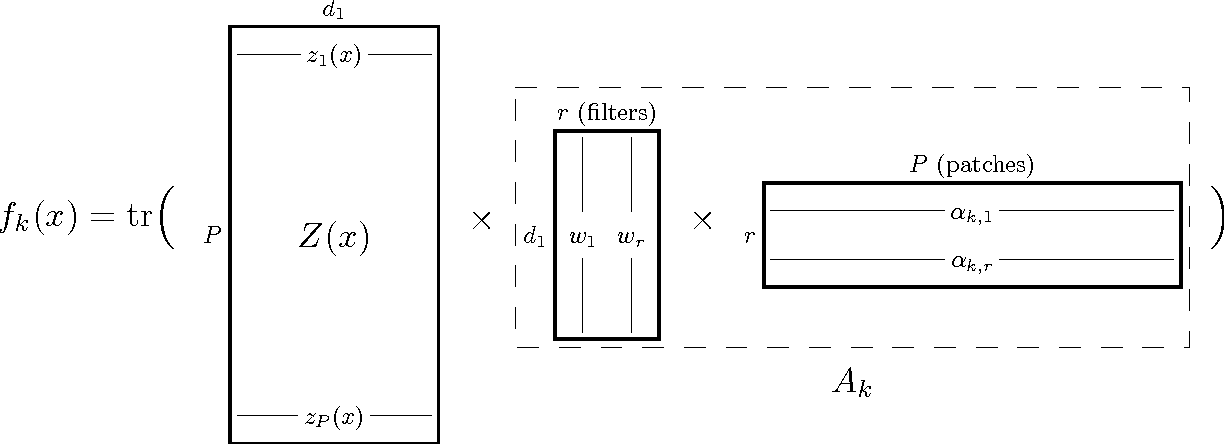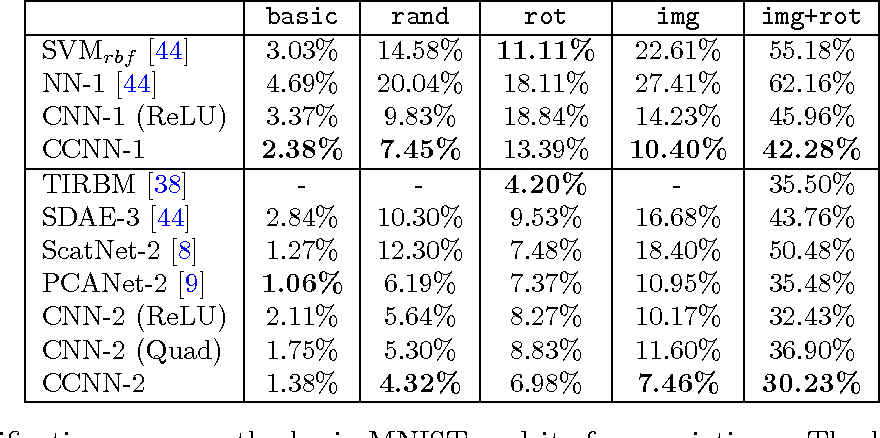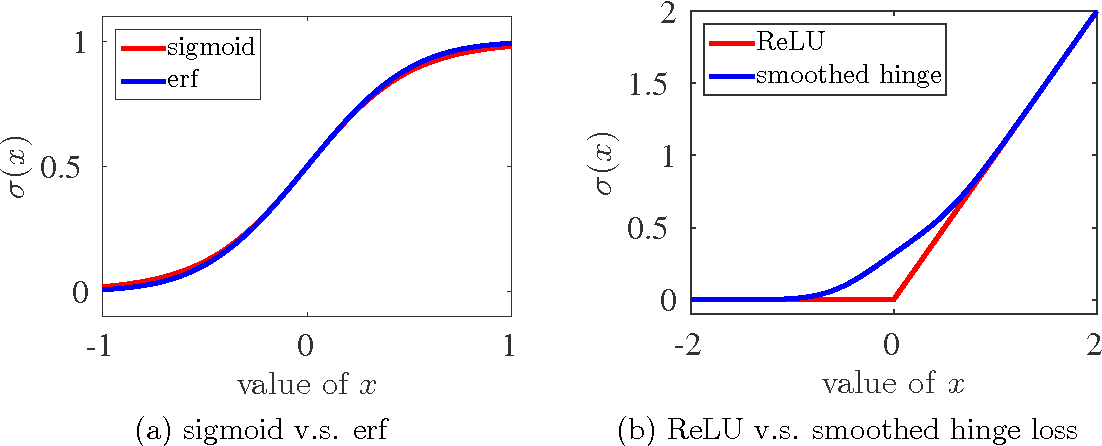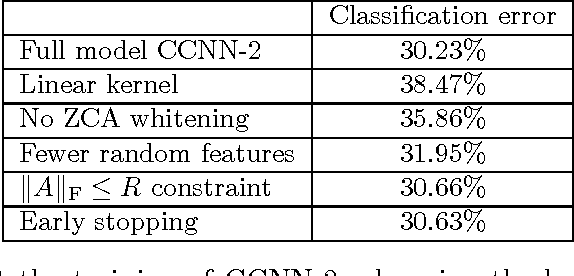We describe the class of convexified convolutional neural networks (CCNNs), which capture the parameter sharing of convolutional neural networks in a convex manner. By representing the nonlinear convolutional filters as vectors in a reproducing kernel Hilbert space, the CNN parameters can be represented as a low-rank matrix, which can be relaxed to obtain a convex optimization problem. For learning two-layer convolutional neural networks, we prove that the generalization error obtained by a convexified CNN converges to that of the best possible CNN. For learning deeper networks, we train CCNNs in a layer-wise manner. Empirically, CCNNs achieve performance competitive with CNNs trained by backpropagation, SVMs, fully-connected neural networks, stacked denoising auto-encoders, and other baseline methods.

* 29 pages
Click to Read Paper and Get Code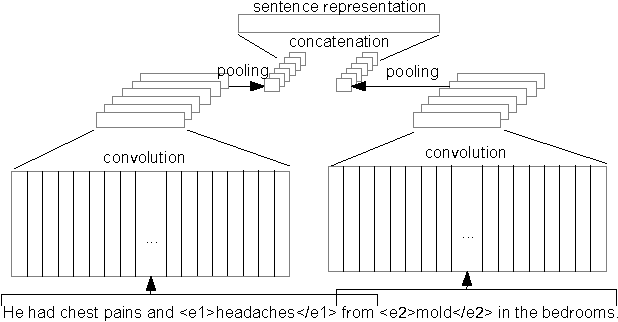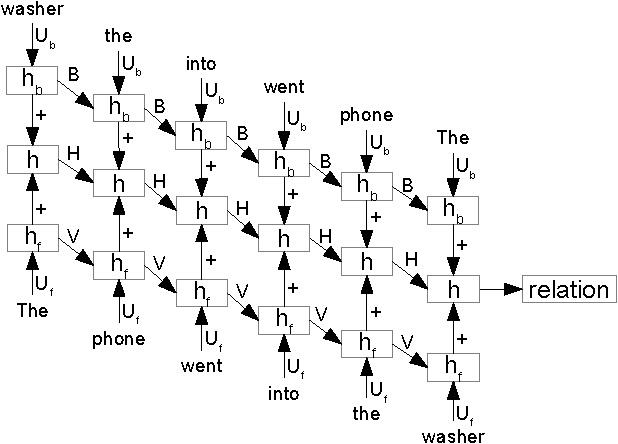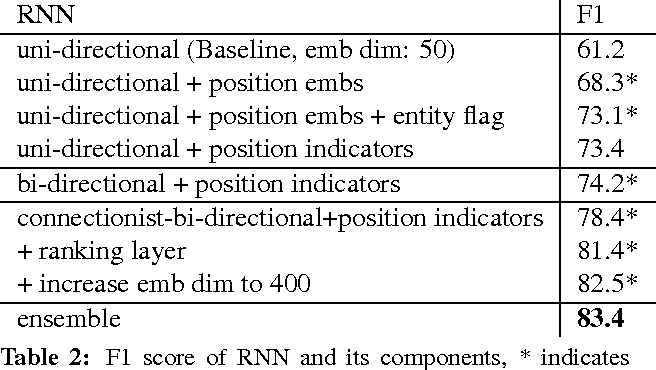This paper investigates two different neural architectures for the task of relation classification: convolutional neural networks and recurrent neural networks. For both models, we demonstrate the effect of different architectural choices. We present a new context representation for convolutional neural networks for relation classification (extended middle context). Furthermore, we propose connectionist bi-directional recurrent neural networks and introduce ranking loss for their optimization. Finally, we show that combining convolutional and recurrent neural networks using a simple voting scheme is accurate enough to improve results. Our neural models achieve state-of-the-art results on the SemEval 2010 relation classification task.

* NAACL 2016
Click to Read Paper and Get Code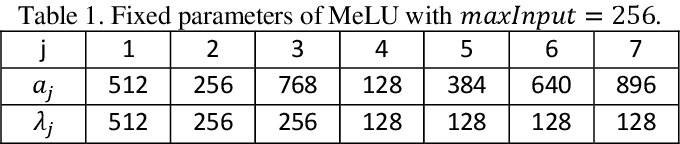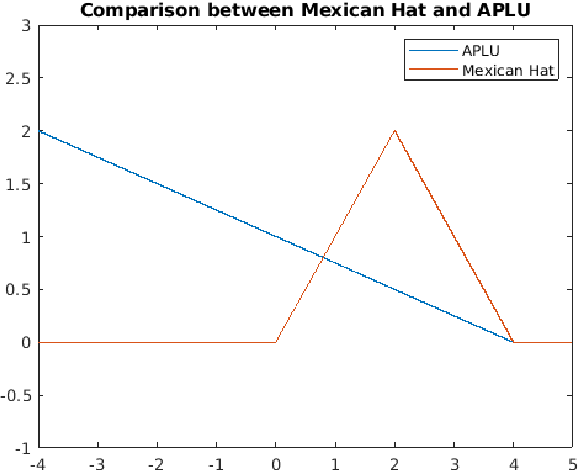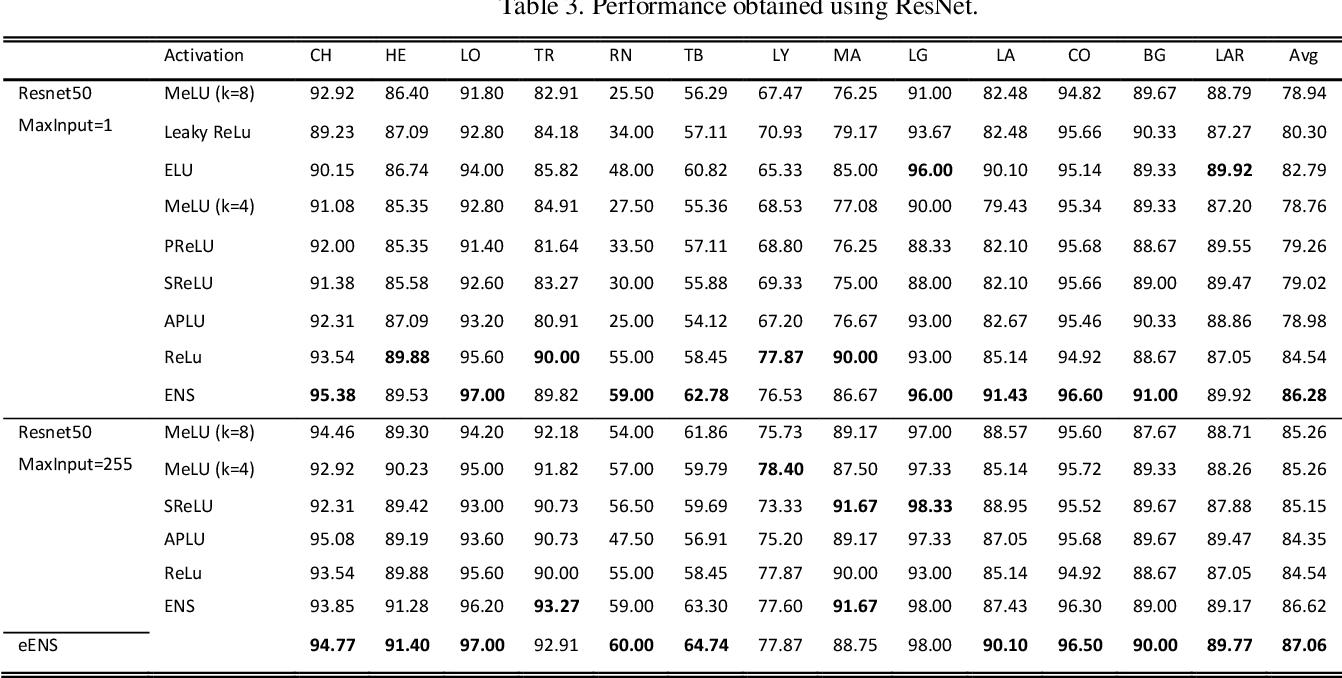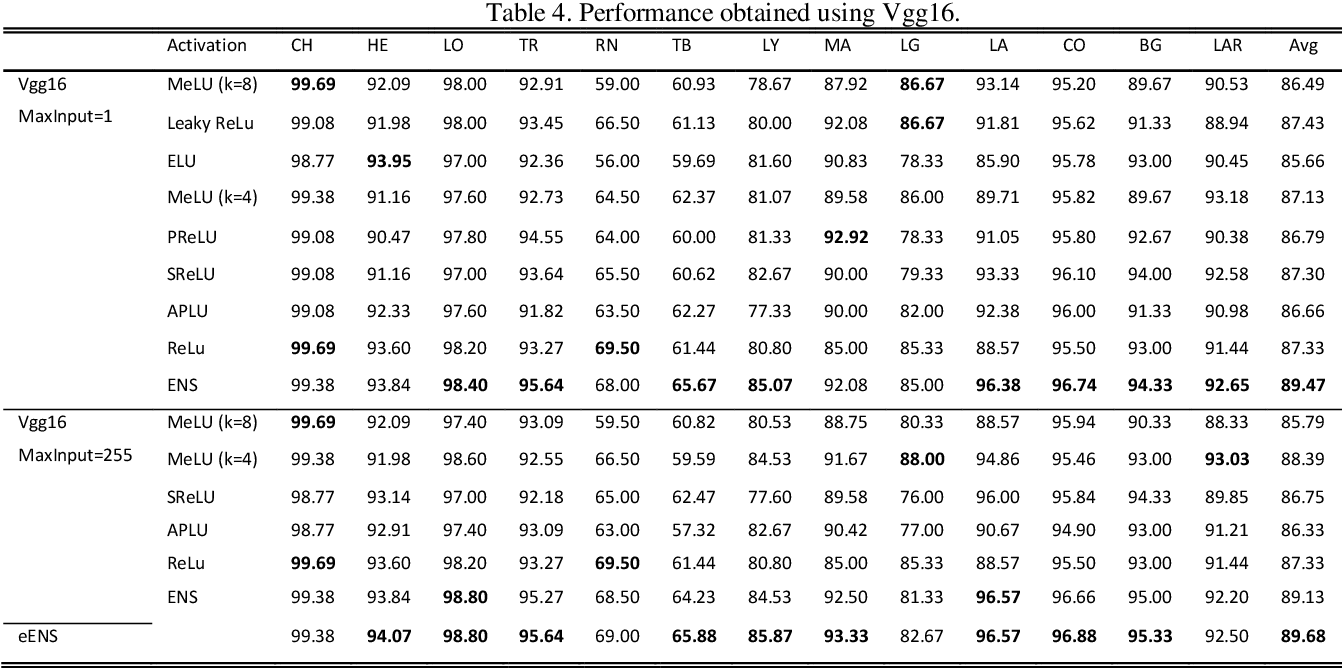Activation functions play a vital role in the training of Convolutional Neural Networks. For this reason, to develop efficient and performing functions is a crucial problem in the deep learning community. Key to these approaches is to permit a reliable parameter learning, avoiding vanishing gradient problems. The goal of this work is to propose an ensemble of Convolutional Neural Networks trained using several different activation functions. Moreover, a novel activation function is here proposed for the first time. Our aim is to improve the performance of Convolutional Neural Networks in small/medium size biomedical datasets. Our results clearly show that the proposed ensemble outperforms Convolutional Neural Networks trained with standard ReLU as activation function. The proposed ensemble outperforms with a p-value of 0.01 each tested stand-alone activation function; for reliable performance comparison we have tested our approach in more than 10 datasets, using two well-known Convolutional Neural Network: Vgg16 and ResNet50. MATLAB code used here will be available at https://github.com/LorisNanni.

Click to Read Paper and Get Code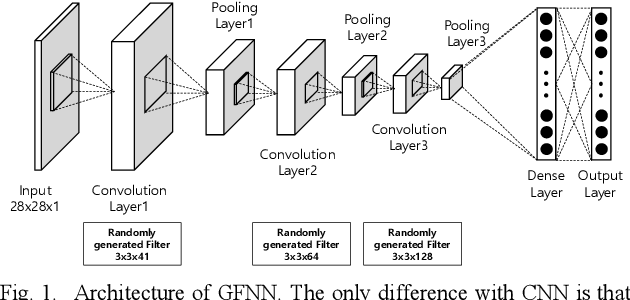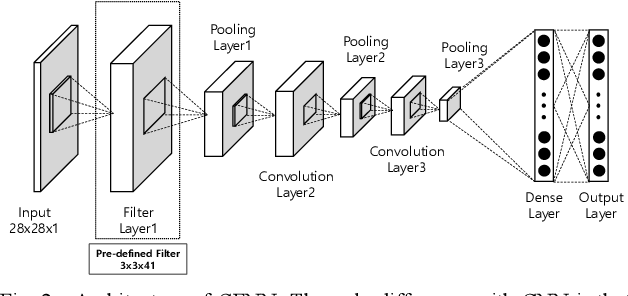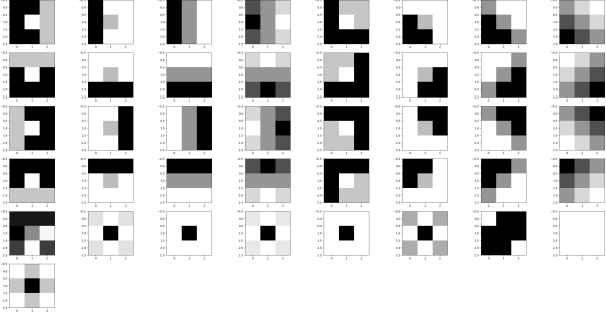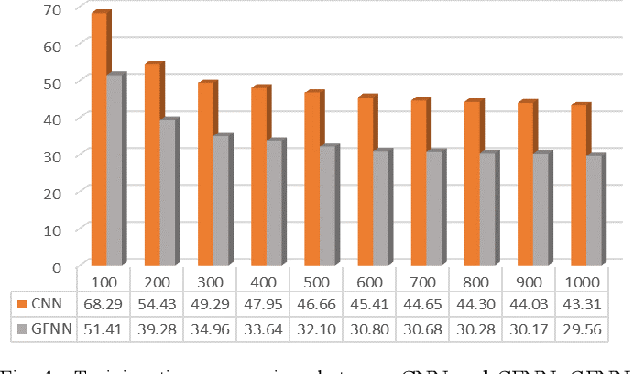We applied pre-defined kernels also known as filters or masks developed for image processing to convolution neural network. Instead of letting neural networks find its own kernels, we used 41 different general-purpose kernels of blurring, edge detecting, sharpening, discrete cosine transformation, etc. for the first layer of the convolution neural networks. This architecture, thus named as general filter convolutional neural network (GFNN), can reduce training time by 30% with a better accuracy compared to the regular convolutional neural network (CNN). GFNN also can be trained to achieve 90% accuracy with only 500 samples. Furthermore, even though these kernels are not specialized for the MNIST dataset, we achieved 99.56% accuracy without ensemble nor any other special algorithms.

* TENCON 2018
* 4 pages, 6 figures
Click to Read Paper and Get Code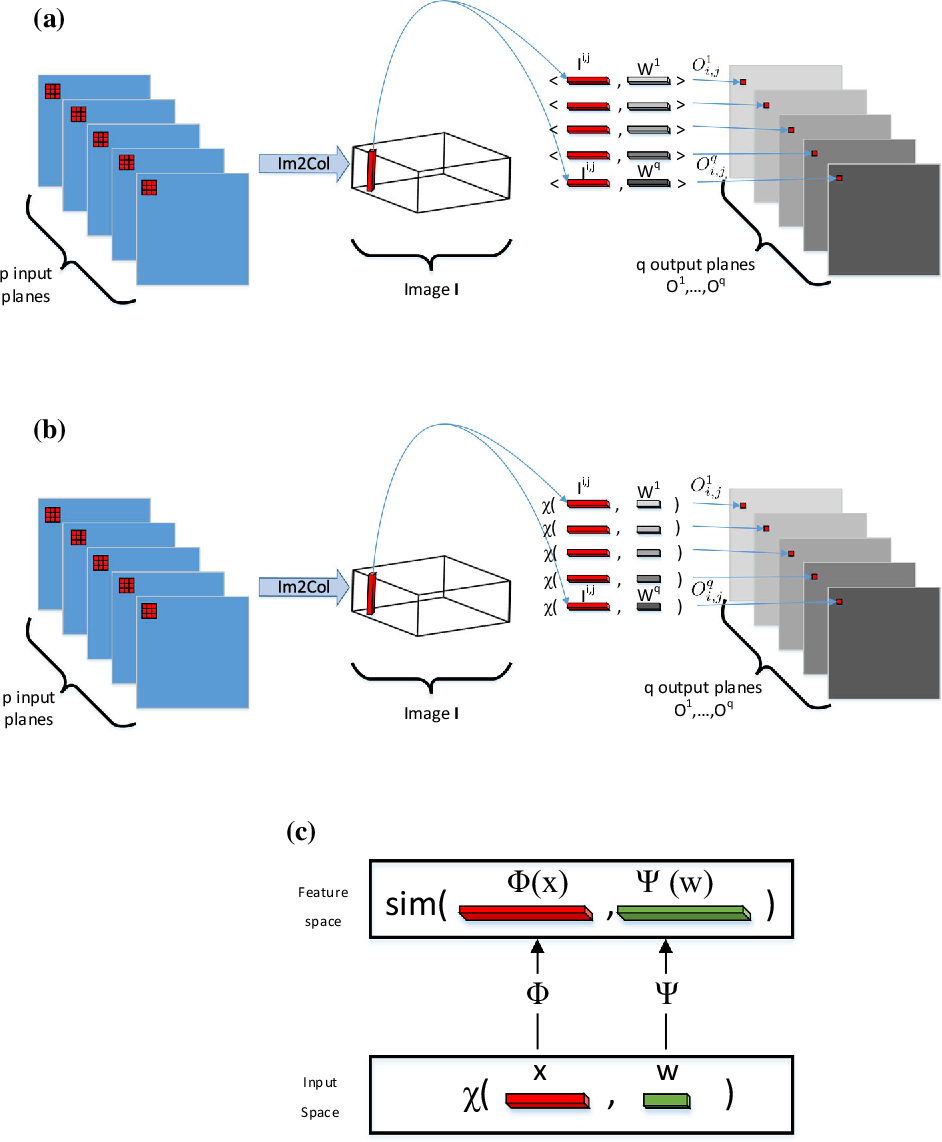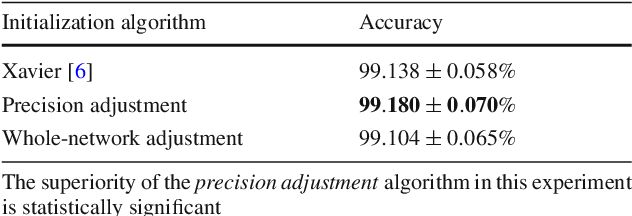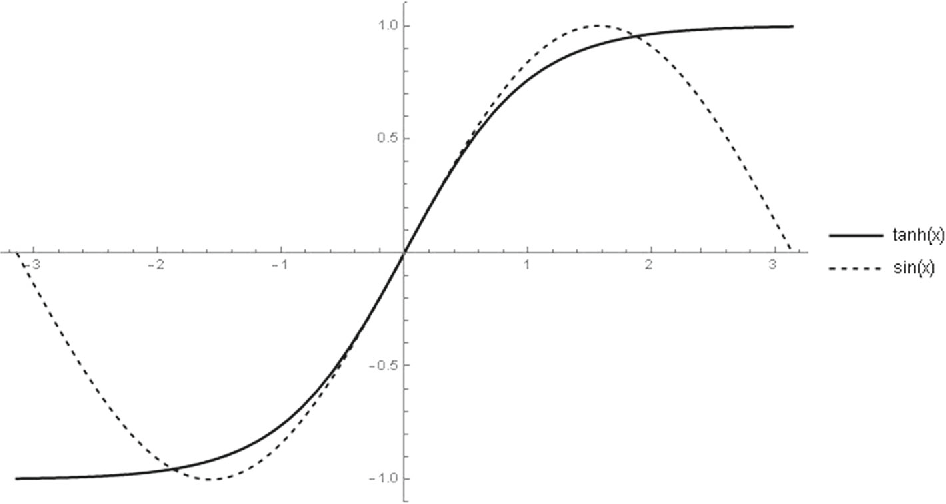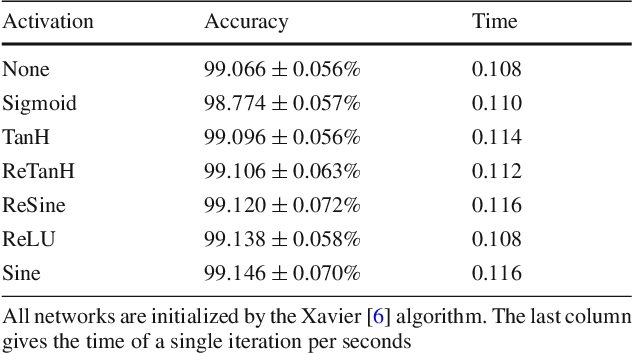Convolutional neural networks have become a main tool for solving many machine vision and machine learning problems. A major element of these networks is the convolution operator which essentially computes the inner product between a weight vector and the vectorized image patches extracted by sliding a window in the image planes of the previous layer. In this paper, we propose two classes of surrogate functions for the inner product operation inherent in the convolution operator and so attain two generalizations of the convolution operator. The first one is the class of positive definite kernel functions where their application is justified by the kernel trick. The second one is the class of similarity measures defined based on a distance function. We justify this by tracing back to the basic idea behind the neocognitron which is the ancestor of CNNs. Both methods are then further generalized by allowing a monotonically increasing function to be applied subsequently. Like any trainable parameter in a neural network, the template pattern and the parameters of the kernel/distance function are trained with the back-propagation algorithm. As an aside, we use the proposed framework to justify the use of sine activation function in CNNs. Our experiments on the MNIST dataset show that the performance of ordinary CNNs can be achieved by generalized CNNs based on weighted L1/L2 distances, proving the applicability of the proposed generalization of the convolutional neural networks.

Click to Read Paper and Get Code
Deep learning has been widely applied and brought breakthroughs in speech recognition, computer vision, and many other domains. The involved deep neural network architectures and computational issues have been well studied in machine learning. But there lacks a theoretical foundation for understanding the approximation or generalization ability of deep learning methods generated by the network architectures such as deep convolutional neural networks having convolutional structures. Here we show that a deep convolutional neural network (CNN) is universal, meaning that it can be used to approximate any continuous function to an arbitrary accuracy when the depth of the neural network is large enough. This answers an open question in learning theory. Our quantitative estimate, given tightly in terms of the number of free parameters to be computed, verifies the efficiency of deep CNNs in dealing with large dimensional data. Our study also demonstrates the role of convolutions in deep CNNs.

Click to Read Paper and Get Code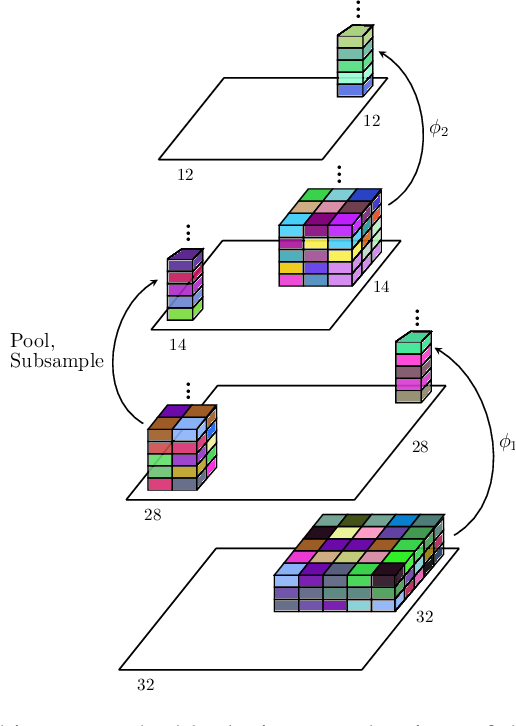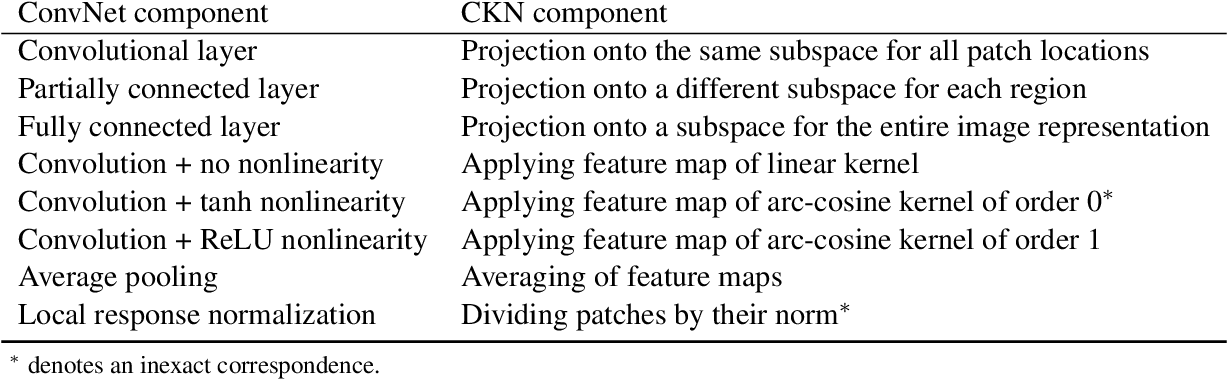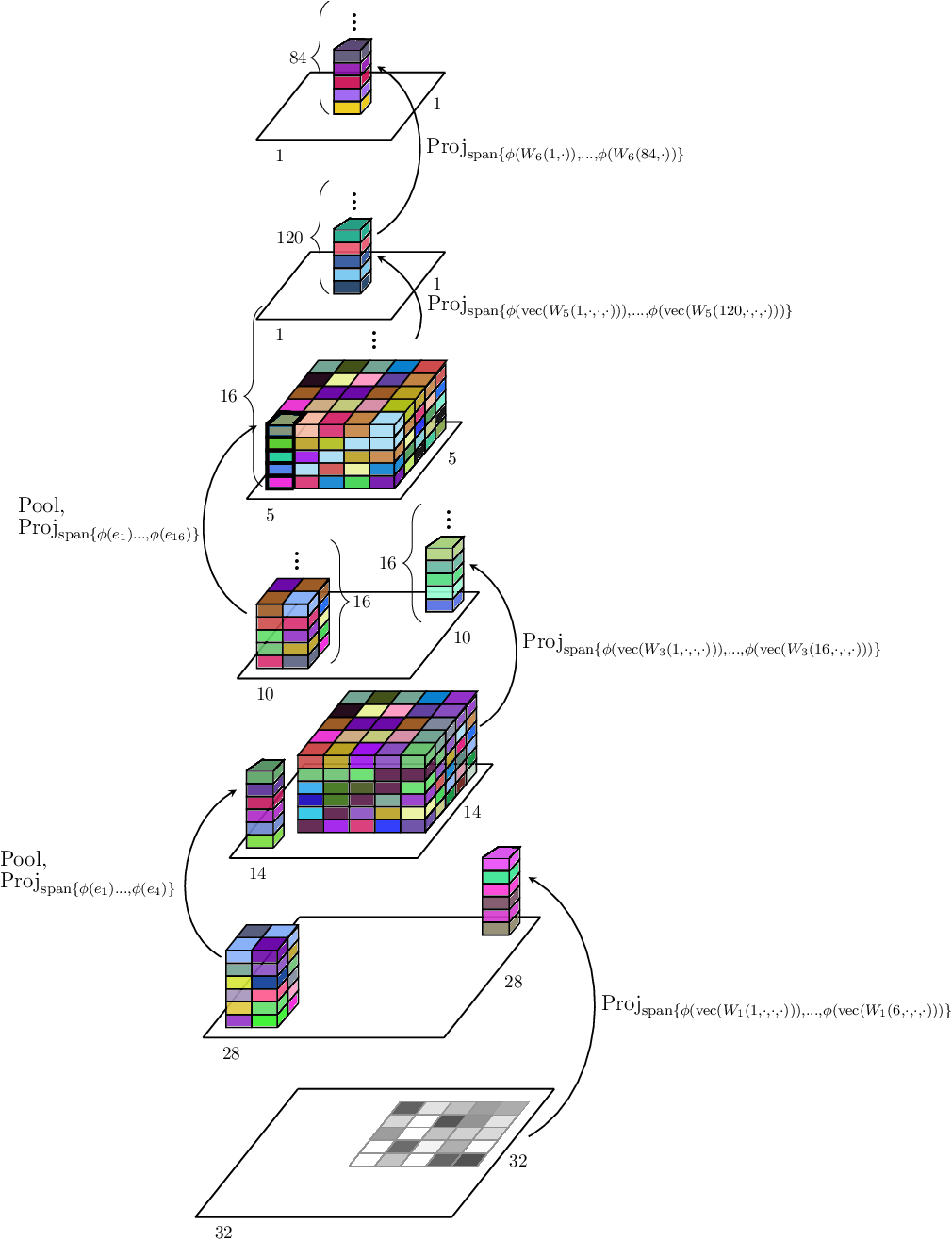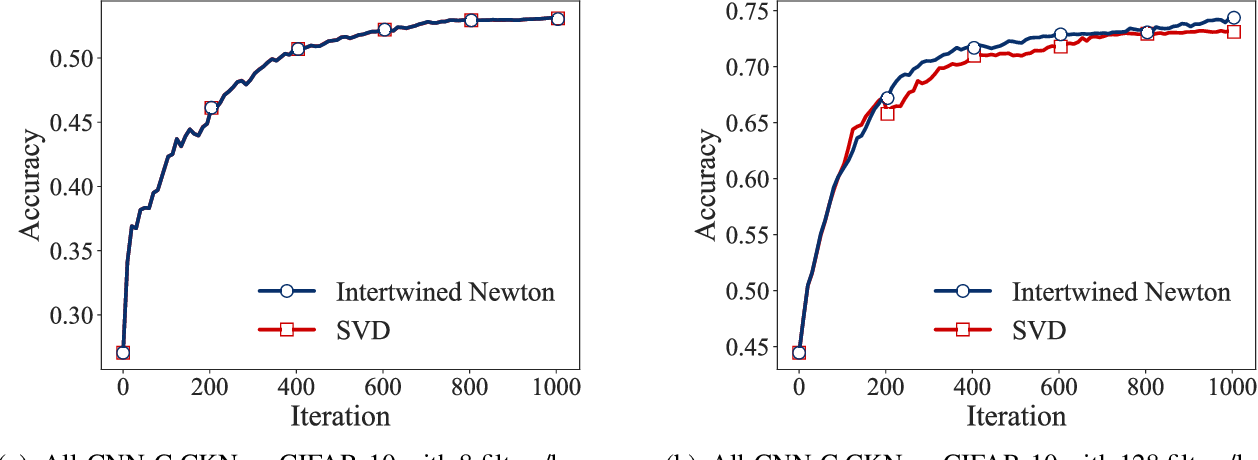Convolutional Neural Networks, as most artificial neural networks, are commonly viewed as methods different in essence from kernel-based methods. We provide a systematic translation of Convolutional Neural Networks (ConvNets) into their kernel-based counterparts, Convolutional Kernel Networks (CKNs), and demonstrate that this perception is unfounded both formally and empirically. We show that, given a Convolutional Neural Network, we can design a corresponding Convolutional Kernel Network, easily trainable using a new stochastic gradient algorithm based on an accurate gradient computation, that performs on par with its Convolutional Neural Network counterpart. We present experimental results supporting our claims on landmark ConvNet architectures comparing each ConvNet to its CKN counterpart over several parameter settings.

Click to Read Paper and Get Code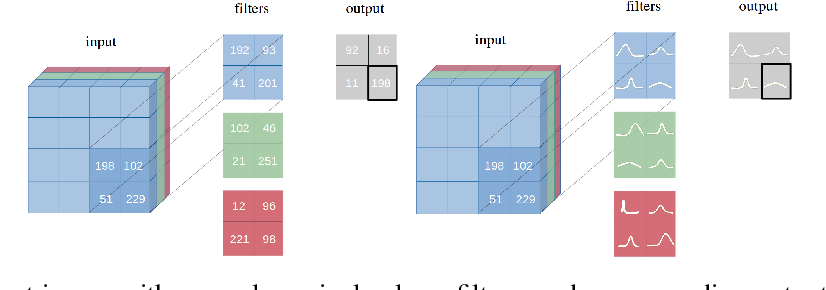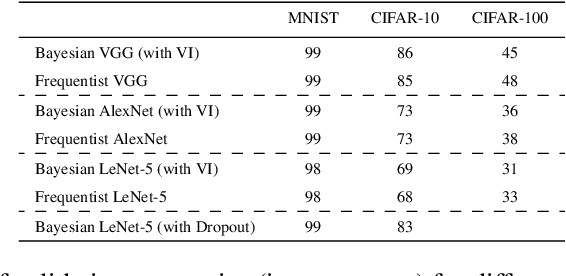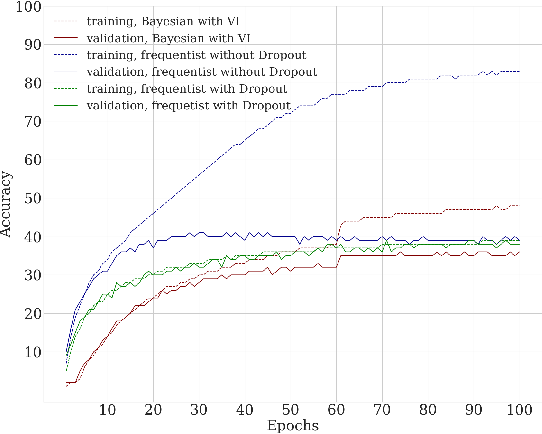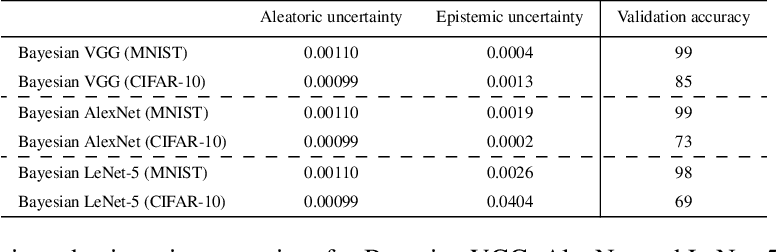We introduce Bayesian Convolutional Neural Networks (BayesCNNs), a variant of Convolutional Neural Networks (CNNs) which is built upon Bayes by Backprop. We demonstrate how this novel reliable variational inference method can serve as a fundamental construct for various network architectures. On multiple datasets in supervised learning settings (MNIST, CIFAR-10, CIFAR-100, and STL-10), our proposed variational inference method achieves performances equivalent to frequentist inference in identical architectures, while a measurement for uncertainties and a regularisation are incorporated naturally. In the past, Bayes by Backprop has been successfully implemented in feedforward and recurrent neural networks, but not in convolutional ones. This work symbolises the extension of Bayesian neural networks which encompasses all three aforementioned types of network architectures now.

* arXiv admin note: text overlap with arXiv:1704.02798 by other authors
Click to Read Paper and Get Code